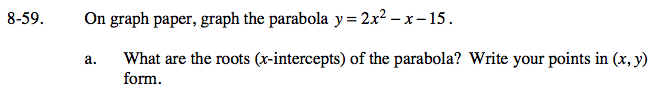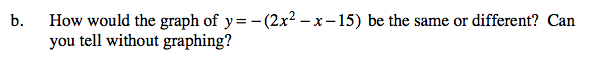Home > GC > Chapter 8 > Lesson 8.1.5 > Problem8-59

8-59.
1. On graph paper, graph the parabola y = 2x2x − 15. 8-59 HW eTool (Desmos). Homework Help ✎

1. What are the roots (x-intercepts) of the parabola? Write your points in (x, y) form.

2. How would the graph of y = −(2x2x − 15) be the same or different? Can you tell without graphing?Use the eTool below to solve each part.
Click the link at right for the full version of the eTool: GC 8-59 HW eTool

Look at the graph above to help you write your points in (x, y) form.

What does multiplying −1 into an equation do to the graph?
Think, how is y = x different from y = −x.